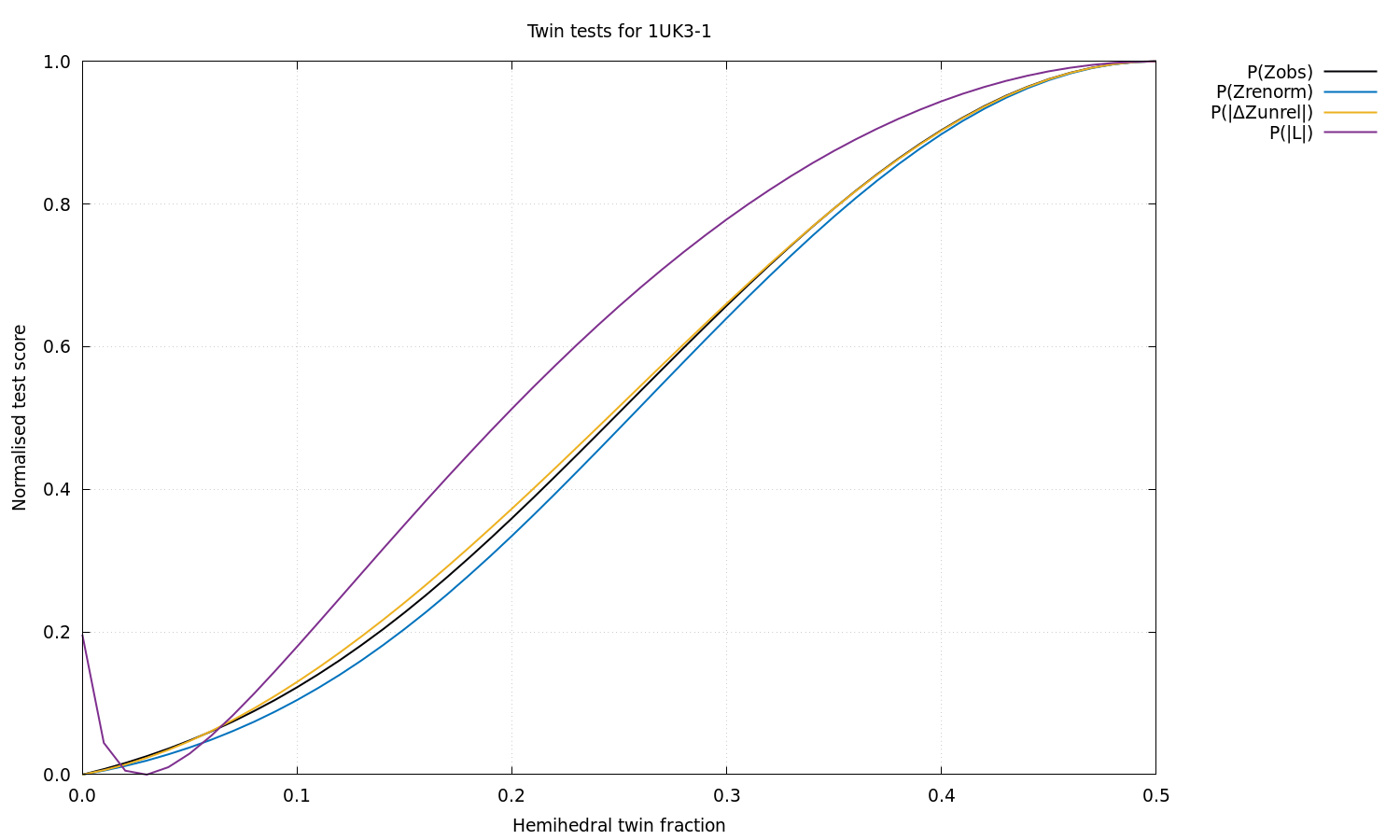### Anisotropy information extracted from the STARANISO log file for 1UK3:

```Processing software:          DENZO(integration)  SCALEPACK(scaling)

Using MTZ column labels:      IMEAN  SIGIMEAN

Unit cell and space group:       49.294   97.033   67.781  90.00 101.92  90.00   'P 1 21 1'
Nominal diffraction range:       48.517  2.403
Input reflection count:           24242

Diffraction cut-off criterion: Local mean I/sd(I) = 1.20

Diffraction limits & principal axes of ellipsoid fitted to diffraction cut-off surface:

2.401         0.9978   0.0000   0.0661       0.982 a* - 0.191 c*
2.349         0.0000   1.0000   0.0000       b*
2.323        -0.0661   0.0000   0.9978      -0.049 a* + 0.999 c*

GoF to ellipsoid (d*):  0.0186    Fraction of surface points fitted:  100.0%  (     3790 /     3790)

Number of unobserved reflections inside ellipsoid:      481
Number of observed reflections inside ellipsoid:      23761
Number of observed reflections outside ellipsoid:         0

Lowest cut-off diffraction limit:
2.583 at reflection  -19    0    3  in direction  -0.988 a* + 0.156 c*

Worst diffraction limit after cut-off:
2.716 at reflection  -18    0    2  in direction  -0.994 a* + 0.110 c*

Best diffraction limit after cut-off:
2.403 at reflection    8    4   23  in direction   0.324 a* + 0.162 b* + 0.932 c*

NOTE that because the cut-off surface is likely to be only very approximately ellipsoidal, in part
due to variations in reflection redundancy arising from the chosen collection strategy, the
directions of the worst and best diffraction limits may not correspond with the reciprocal axes,
even in high-symmetry space groups (the only constraint being that the surface must have point
symmetry at least that of the Laue class).

Fraction of data inside cut-off surface:                           98.0%  (    23761 /    24242)

Fraction of surface truncated by detector edges:                   68.0%  (     1134 /     1668)

WARNING: Diffraction of the input data has probably been truncated due to an inappropriate
(an)isotropic diffraction cut-off applied in previous processing, or the diffraction pattern may
have extended beyond the edges of the detector.  In the latter case consider the possibilities of
either moving the detector closer or swinging it out, having carefully checked in the former case
that this will not create a risk of spot overlap.

Fraction of total surface above threshold truncated by cusp(s):     0.0%  (        0 /     1668)

Scale:                       1.08137E+00    [ = factor to place Iobs on same scale as Iprofile/100.]
Beq:                               34.54    [ = equivalent overall isotropic B factor on Fs.]

B11      B22      B33      B23      B31      B12
Delta-B tensor:                               5.34     2.51    -7.85     0.00     5.82     0.00

NOTE: The delta-B tensor is the overall anisotropy tensor on Fs after subtraction of Beq from its
diagonal elements (so trace = 0).

Eigenvalues (E) & eigenvectors of overall anisotropy (B) tensor on Fs:

42.07         0.9354   0.0000   0.3536       0.976 a* + 0.219 c*
37.05         0.0000   1.0000   0.0000       b*
24.49        -0.3536   0.0000   0.9354      -0.252 a* + 0.968 c*

The eigenvalues and eigenvectors of the overall B tensor are the squares of the lengths and the
directions of the principal axes of the ellipsoid that represents the tensor.

Delta-B eigenvalues:                     7.54     2.51   -10.05

The delta-B eigenvalues are the eigenvalues of the overall anisotropy tensor after subtraction of
Beq (so sum = 0).

Angle & axis of rotation of diffraction-limit ellipsoid relative to anisotropy tensor:

16.92         0.0000   1.0000   0.0000

Anisotropy ratio:             0.509    [ = (Emax - Emin) / Beq ]
Fractional anisotropy:        0.256    [ = sqrt(1.5 Sum_i (E_i - Beq)^2 / Sum_i E_i^2) ]

Eigenvalues & eigenvectors of mean I/sd(I) anisotropy tensor:

1.85         0.9991   0.0000  -0.0422       0.947 a* - 0.323 c*
2.34         0.0000   1.0000   0.0000       b*
2.75         0.0422   0.0000   0.9991       0.032 a* + 0.999 c*

Eigenvalues & eigenvectors of weighted CC_1/2 anisotropy tensor:

0.281         0.9973   0.0000   0.0734       0.983 a* - 0.182 c*
0.307         0.0000   1.0000   0.0000       b*
0.320        -0.0734   0.0000   0.9973      -0.054 a* + 0.999 c*

Eigenvalues & eigenvectors of mean K-L divergence anisotropy tensor:

0.613         1.0000   0.0000   0.0000       0.962 a* - 0.273 c*
0.735         0.0000   1.0000   0.0000       b*
0.834         0.0000   0.0000   1.0000       c*

Ranges of local mean I/sd(I), local weighted CC_1/2, local mean K-L divergence and D-W factor [= exp(-4 pi^2 s~Us)]:

ISmean  CChalf  KLdive  DWfact
0   Grey                 Unobservable*
1   Blue                  Observable*
2   Red|Pink:9    1.20  0.3000   1.874  0.0762
3   Orange        5.88  0.9480   3.101  0.1609
4   Yellow       14.09  0.9815   4.005  0.2988
5   Green        19.64  0.9846   4.343  0.4884
6   Cyan         22.85  0.9857   4.691  0.7028
7   Magenta      25.97  0.9870   5.754  0.8900
8   White        27.03  0.9894   6.689  0.9922

* Refer to GLOSSARY for explanation of terminology.

The cut-off surface uses a different color scheme:
Unmeasured points are blue (inside the fitted surface) or cyan (outside).
Unobserved points are red (in) or green (out).
Observed points are orange (in) or white (out).
The fitted surface is magenta.

Anisotropic S/N ratio:         4.86    [ = max_h | exp(4 pi^2 s~_h delta(B) s_h) - 1 | <I_h/sd(I_h)> ]

The 'anisotropic S/N ratio', unlike the 'anisotropy ratio' or the 'fractional' anisotropy shown
above, in addition to the anisotropy of the B tensor, takes both the diffraction and the local mean
I/sd(I) into account.

Estimated twin fraction from K-L divergence of observed acentric Z probability (before, after):  0.00  0.00

Estimated twin fraction from K-L divergence of posterior acentric Z probability:                 0.00

Estimated twin fraction from K-L divergence of unrelated acentric |delta-Z| probability:         0.00

Padilla & Yeates L test for twinning, acentric moments of |L|:
<|L|> (normal = .500; perfect twin = .375):  0.486
<L^2> (normal = .333; perfect twin = .200):  0.313

Estimated twin fraction from K-L divergence of |L| probability:                                  0.03

```

### Normalised scores from a panoply of hemihedral twinning tests.

For each test, the most likely hemihedral twin fraction based on the data is the one corresponding to the lowest normalised score (i.e. like the R value).  For all the distributional tests (i.e. those of the form P(x)), the raw score before normalisation is the Kullback-Leibler divergence from the theoretical to the observed probability density.  For the non-distributional Britton and Fisher & Sweet tests, it's the count of extra negative intensities obtained by detwinning the data using each possible twin fraction.  Note: click image to enlarge.Reference